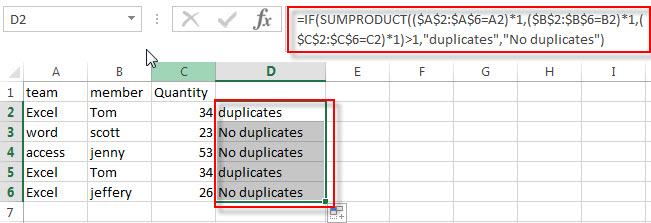# Find Duplicate Rows

In the previous post, we talked that how to highlight duplicate rows with conditional formatting feature in excel. And this post will teach you how to find duplicate rows with an excel formula.

If you want to check the entire row that duplicated or not, if True, then returns “duplicates” value, otherwise, returns “no duplicates”. How to achieve it? You can create a formula based on the IF function and the SUMPRODUCT function.

Assuming that you want to find or check duplicate rows in range A2:C6, then you can write down the following formula:

`=IF(SUMPRODUCT((\$A\$2:\$A\$6=A2)*1,(\$B\$2:\$B\$6=B2)*1,(\$C\$2:\$C\$6=C2)*1)>1,"duplicates","No duplicates")`You can enter the above formula into the formula box in Cell D2, then press Enter. You will see that if there are same rows in the range, it will display “duplicates”, or it will display “No duplicates”.

Last, you can drag the AutoFill Handle down to the rest cells in Column D to check duplicate rows.

### Related Functions

• Excel SUMPRODUCT function
The Excel SUMPRODUCT function multiplies corresponding components in the given one or more arrays or ranges, and returns the sum of those products.The syntax of the SUMPRODUCT function is as below:= SUMPRODUCT (array1,[array2],…)…
• Excel IF function
The Excel IF function perform a logical test to return one value if the condition is TRUE and return another value if the condition is FALSE. The IF function is a build-in function in Microsoft Excel and it is categorized as a Logical Function.The syntax of the IF function is as below:= IF (condition, [true_value], [false_value])….

### Related Posts

• Highlight Rows
You will learn that how to change the color of the entire rows if the value of cells in a specified column meets your conditions, such as, if the value of cells is equal to or greater than a certain number or text values, then excel should be highlight entire rows or change a row color as you need.…
• Highlight Duplicate Rows
this post will talk that how to highlight entire rows that are duplicates in excel 2016, 2013 or lower version. Or how to change the background color of duplicate rows..…
• Highlight duplicate values
this post will teach you how to highlight duplicate values in the range of cells in excel. Normally, you may be need to identify duplicate values with a range of cells in Excel. And there is one of the fasted way that is using conditional formatting feature in Microsoft Excel……
• Combine Duplicate Rows and Sum the Values
This post will teach you how to combine duplicate rows and sum the corresponding values or calculate numbers in specific column in excel. And how to merge duplicate rows and then sum the values with VBA macro in Excel..…